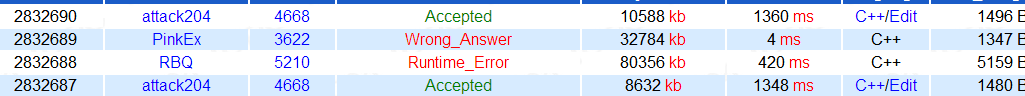# BZOJ4668: 冷战(并查集)

Time Limit: 10 Sec  Memory Limit: 256 MB
Submit: 538  Solved: 269
[Submit][Status][Discuss]

## Description

1946 年 3 月 5 日，英国前首相温斯顿·丘吉尔在美国富尔顿发表“铁

Reddington 是美国的海军上将。由于战争局势十分紧张，因此他需要

Reddington 得到了苏联的修建日程表，并且他需要时刻关注着某两个军工

M 个操作，操作分为两类：
• 0 u v，这次操作苏联会修建一条连接 u 号军工厂及 v 号军工厂的铁

• 1 u v， Reddington 需要知道 u 号军工厂及 v 号军工厂最早在加入第

## Input

u, v 异或上上一次询问的答案。一开始这个值为 0。
1 ≤ N, M ≤ 500000，解密后的 u, v 满足1 ≤ u, v ≤ N, u不等于v

5 9
0 1 4
1 2 5
0 2 4
0 3 4
1 3 1
0 7 0
0 6 1
0 1 6
1 2 6

0
3
5

## Source#include<cstdio>
#include<vector>
#include<algorithm>
#define Pair pair<int, int>
#define MP(x, y) make_pair(x, y)
using namespace std;
const int MAXN = 500001, INF = 1e9 + 10;
char c = getchar(); int x = 0, f = 1;
while(c < '0' || c > '9'){if(c == '-')f = -1; c = getchar();}
while(c >= '0' && c <= '9') x = x * 10 + c - '0', c = getchar();
return x * f;
}
int N, M;
int fa[MAXN], h[MAXN], deep[MAXN], mx[MAXN], lastans = 0, siz[MAXN];
int find(int x) {
if(x == fa[x]) return x;
else {
int f = find(fa[x]);
deep[x] = deep[fa[x]] + 1;
return f;
}
}
void unionn(int x, int y, int tim) {
int fx = find(x), fy = find(y);
if(fx == fy) return;
if(siz[fx] > siz[fy]) swap(fx, fy);
fa[fx] = fy; siz[fy] += siz[fx];
mx[fx] = tim;
}
int query(int x, int y) {
int fx = find(x), fy = find(y);
if(fx != fy) return 0;
int ans = 0;
while(x != y) {
if(deep[x] < deep[y]) swap(x, y);
ans = max(ans, mx[x]);
x = fa[x];
}
return ans;
}
int main() {
#ifdef WIN32
//freopen("a.in", "r", stdin);
#endif
for(int i = 1; i <= N; i++) fa[i] = i, siz[i] = 1;
int id = 0;
for(int i = 1; i <= M; i++) {
}